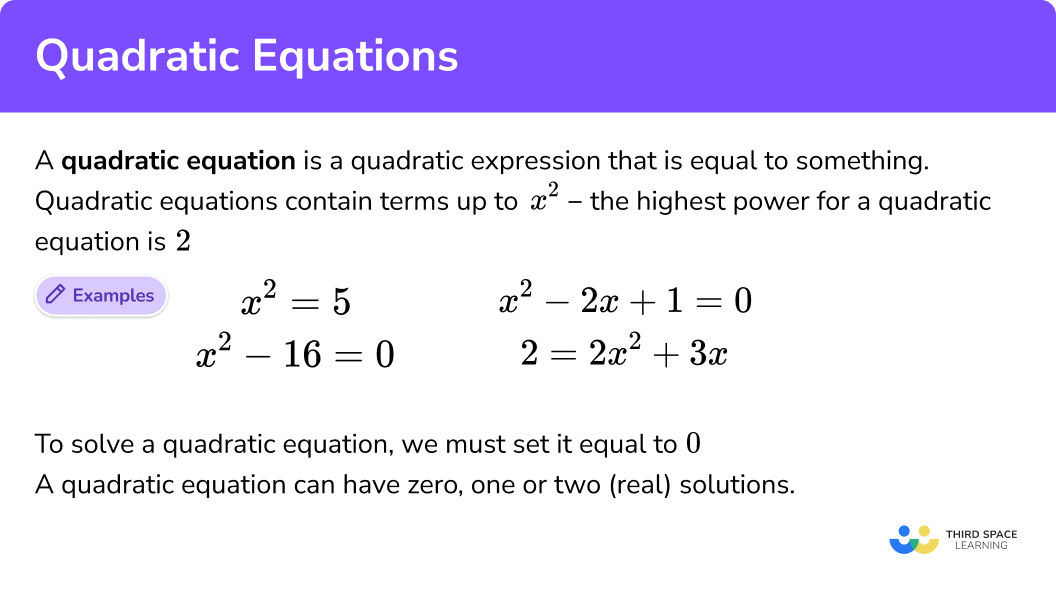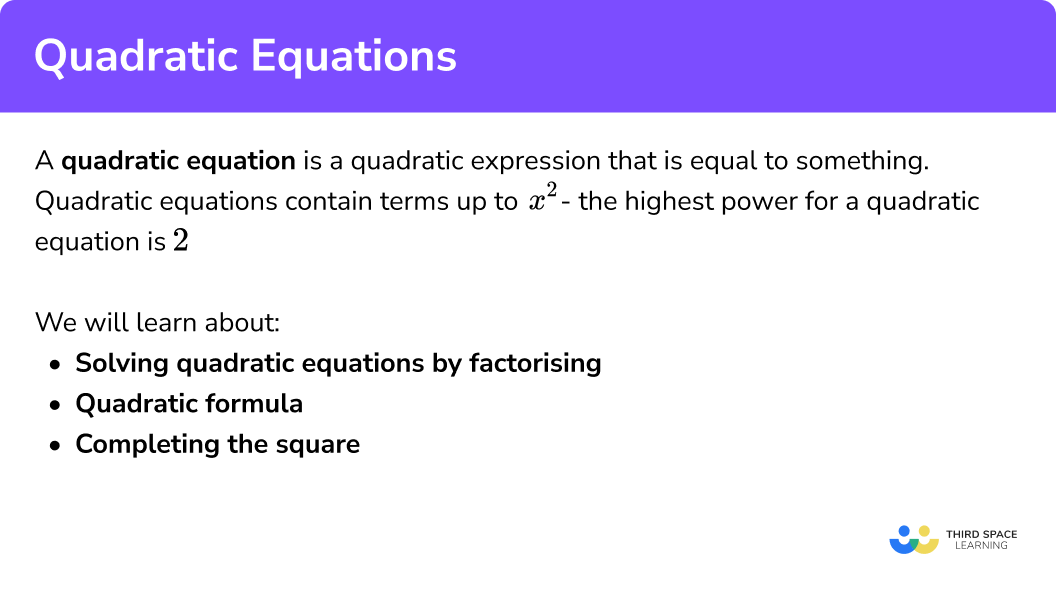Here we will learn about the quadratic equation and how to solve quadratic equations using four methods: factorisation, using the quadratic equation formula, completing the square and using a graph.

There are also quadratic equation worksheets based on Edexcel, AQA and OCR exam questions, along with further guidance on where to go next if you’re still stuck.

## What is a quadratic equation?

A quadratic equation is a quadratic expression that is equal to something.

Quadratic algebraic equations are equations that contain terms up to x2; the highest power for a quadratic equation is 2.

Quadratic equations are a type of polynomial equation because they consist of two or more algebraic terms.

To solve a quadratic equation it must equal 0.

Example:

\begin{aligned} x^{2}&=5\\ x^{2}-2x+1&=0\\ 2x^{2}+3x-2&=0\ \end{aligned}

A quadratic equation can have zero, one or two (real) solutions.

The general example of a quadratic equation formula is written as:

$a{x}^2+bx+c=0$

a is coefficient (number in front) of the x2 term

b is coefficient (number in front) of the x term

c is the constant term (number on its own)

At GCSE the solutions to polynomial equations such as quadratics will always give real numbers but they can be either irrational and rational numbers.

### What is a quadratic equation?## How to solve a quadratic equation

In order to solve a quadratic equation we must first check that it is in the form:

$a x^{2}+b x+c=0$

If it isn’t, we will need to rearrange the equation.

Example:

### Explain how to solve a quadratic equation## Ways of solving quadratic equations

Although the most common way of solving quadratic equations is through factorising, there are in fact three other ways to solve them as well. We will explore how to solve the same quadratic equation in each of the four ways.

$x^{2}-2x-24=0$

Step-by-step guide: Solving equations

### a) Factorisation

Example of solving a quadratic equation by factorisation:

Solve

$x^{2}-2x-24=0$

$(x-6)(x+4)=0$ \begin{aligned} x-6&=0 \quad &x+4&=0\\ x&=6 \quad &x&=-4 \end{aligned}

Step-by-step guide: Solving quadratic equations by factorising

$x=\frac{-b\pm\sqrt{b^2-4ac}}{2a}$

Solve

$x^{2}-2x-24=0$

The standard form of a quadratic equation is given by

$ax^{2}+bx+c=0$

$a=1, \quad b=-2, \quad c=-24$ $x=\frac{-(-2)\pm\sqrt{(-2)^2-4(1)(-24)}}{2(1)}$ $x=6 \quad and \quad x=-4$

### c) Completing the square (H)

Example of solving a quadratic equation by completing the square

Solve

$x^{2}-2x-24=0$

$(x-1)^{2}-25=0$

$x=6\quad and \quad x=-4$

Step-by-step guide: Completing the square

Solve

$x^{2}-2x-24=0$

The real roots/solutions are shown where the graph crosses the horizontal x-axis.

$x=6\qquad and \qquad x=-4$

### Example 1: quadratic equation – solve by factorising

Example of solving a quadratic equation by factorising (also known as factoring).

To solve

$x^{2}-2x-24=0$

1. Fully factorise the quadratic equation.

We can factorise a quadratic into two brackets when it is in the form ax2 + bx + c or when two square numbers (perfect squares) are subtracted from each other in the form a2 − b2, also known as the difference of two squares.

$x^{2}-2x-24=0$

Factors
1, 24
2, 12
3, 8
4, 6

\begin{aligned} -6+4&=-2\\ -6\times 4&=-24 \end{aligned}

$(x-6)(x+4)=0$

2 Set each bracket equal to 0.

$x-6=0\qquad \qquad x+4=0$

3 Solve each equation to find x.

\begin{aligned} x-6&=0 \quad &x+4&=0\\ x&=6 \quad &x&=-4 \end{aligned}

We can check that our solution is correct by substituting it into the original equation.

### Example 2: quadratic equation – solve by using the quadratic formula

To solve

$x^{2}-2x-24=0$

1. Identify the a, b and c.

$a=1, \quad b=-2, \quad c=-24$

2 Substitute the these values into the quadratic formula.

The

$\sqrt{b^{2}-4 a c}$

part of the quadratic formula is called the discriminant and tells us how many real roots (solutions) the equation has.

$x=\frac{-b\pm\sqrt{b^2-4ac}}{2a}$
$x=\frac{-(-2)\pm\sqrt{(-2)^2-4(1)(-24)}}{2(1)}$

Using brackets will help to make the calculation clear.

3 Use a calculator to solve the equation with a +, and then with a −.

\begin{aligned} x&=\frac{-(-2)+\sqrt{(-2)^2-4(1)(-24)}}{2(1)} \quad &x&=\frac{-(-2)-\sqrt{(-2)^2-4(1)(-24)}}{2(1)}\\ x&=6 \quad &x&=-4 \end{aligned}

We can check that our solution is correct by substituting it into the original equation.

### Example 3: quadratic equation – solve by completing the square

Example of solving a quadratic equation by completing the square.

To solve

$x^{2}-2x-24=0$

1. Complete the square to rewrite the quadratic equation in the form a(x + d)2 + e = 0.

$(x-1)^{2}-25=0$

2 Rearrange the equation to work out the unknown variable (x).

The opposite of − 25 is + 25, so + 25 to both sides of the equation.

The opposite of squaring is square root, so take the square root of the left hand side, and the right hand side.

Remember a square root has a positive and a negative solution, so use the ± sign.

3 The square root has a + and – answer, write down both versions of the calculation to find the two solutions of x.

\begin{aligned} x&=\sqrt{25}+1 \quad &x&=-\sqrt{25}+1\\ x&=5+1 \quad &x&=-5+1\\ x&=6 \quad &x&=-4 \end{aligned}

We can check that our solution is correct by substituting it into the original equation.

### Example 4: quadratic equation – solve by drawing a graph

We can plot a quadratic equation to form a quadratic graph to help us to solve it.

We can substitute values for x into quadratic function to produce values for y. When we plot these values on an x, y grid we get a special ‘U’ shaped curve called a parabola.

Example of solving a quadratic equation by drawing the graph:

To solve

$x^{2}-2x-24=0$

we need to:

2 The solutions/roots of equation are where the graph crosses the x -axis (when y =0)

We know that to solve a quadratic it must be equal to 0

Because x2 – 2x – 24 = 0 we are looking for the values of x that when substituted into the equation will give us a y value of 0

On the graph the coordinates for x where y = 0 are given where the graph crosses the x axis

$x=6 \quad \quad x=-4$

So the solutions or roots of the equation

$x^{2}-2 x-24=0$

are

$x=6 \quad and \quad x=-4$

We can check that our solution is correct by substituting it into the original equation.

Step-by-step guide: Solving quadratic equations graphically

Solve the following by either using factorising, the quadratic formula, or completing the square. What would the quadratic graph look like?

1. Solve:

{x}^2+x-6=0{x}^2+x-6=0

can be factorised as

(x+3)(x-2)=0

By setting each bracket equal to zero and solving, we get the required solutions.2. Solve:

{x}^2-4x-1=0

x=-0.236 \; (3 s.f) \quad x=-4.23 \; (3 s.f)x=0.236 \; (3 s.f) \quad x=4.23 \; (3 s.f)x=-0.236 \; (3 s.f) \quad x=4.23 \; (3 s.f)x=0.236 \; (3 s.f) \quad x=-4.23 \; (3 s.f)This quadratic equation cannot be factorised so we need to use the quadratic formula with a=1, \; b=-4 and c=-1 . Be careful when substituting negative numbers; the signs are important.3. Solve:

2{x}^2-7x+6=02{x}^2-7x+6=0

can be factorised as

(2x-3)(x-2)=0

By setting each bracket equal to zero and solving, we get the required solutions.4. Solve:

3{x}^2+5x-2=0

x=2 \quad x=\frac{1}{3}=0.333 \; (3.5 sf)x=-2 \quad x=\frac{1}{3}=0.333 \; (3.5 sf)x=2 \quad x=-\frac{1}{3}=-0.333 \; (3.5 sf)x=-2 \quad x=-\frac{1}{3}=-0.333 \; (3.5 sf)3{x}^2+5x-2=0

can be factorised as

(3x-1)(x+2)=0

By setting each bracket equal to zero and solving, we get the required solutions.### Quadratic equation questions for GCSE

1. (a)  Factorise

x^{2}-x-30

(2 marks)

(x + 5) or (x – 6)

(1)

(x + 5) (x – 6)

(1)

1. (b)  Hence or otherwise solve the equation

x^{2}-x-30=0

(1 mark)

x = -5  or  x = 6

(1)

2.  Solve

2 x^{2}+10 x+12=0

(3 marks)

(2x + 4)(x + 3)

(1)

x = -3

(1)

x = -2

(1)

### Did you know?

• Did you know that Al-Khwarizmi (Abu Ja’far Muhammad ibn Musa al-Khwarizmi) was one of the first people in history to write about algebra? He lived in Baghdad around 780 to 850 AD and his book “Hisab Al-jabr w’al-muqabala” is where we get the word ‘algebra’ (meaning ‘restoration of broken parts’).

• Did you know the ancient Babylonians could solve quadratic equations using a method equivalent to the quadratic formula, despite not using algebraic notation!
• Did you know that the ancient Greek mathematician Euclid used geometric methods to solve quadratic equations way back in 300BC! His book, The Elements, is one of the most studied books in human history.

The history of mathematics is amazing!

## Learning checklist

You have now learned how to:

• Solve quadratic equations algebraically by factorising